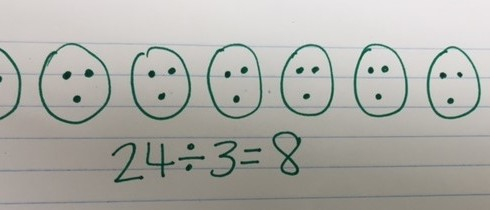## Author Shanna Uhe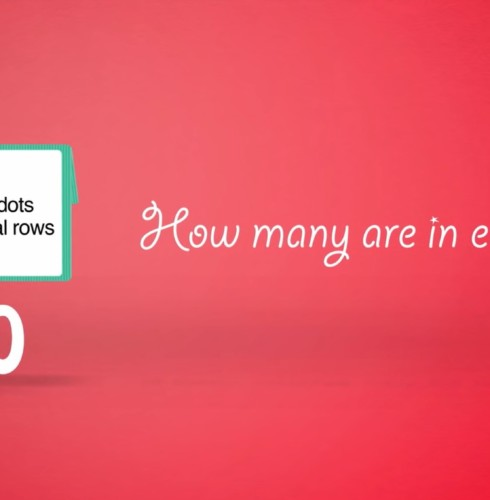For information on using multiplication to divide efficiently and accurately, visit the Origo 1 Minute Math Video: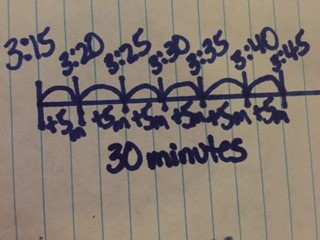To solve story problems involving elapsed time, encourage students to draw open number lines to help students conceptually understand the concept. This could prevent common misconceptions students may have when determining elapsed time. Make sure to include different types of…Telling a time is an important concept for everyday life. It’s also one that students some time struggle with if they don’t understand how a clock works. In 3rd grade, students are expected to: • Tell and write time to…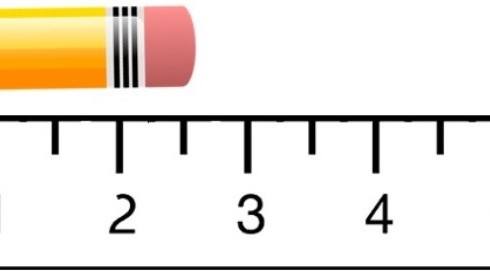Students in 3rd grade are expected to measure length to the nearest whole inch, half inch and quarter inch. In order for students to be successful in measuring length and representing that length as fractions, consider using paper rulers to…Students in 3rd grade are expected to measure length to the nearest whole inch, half inch and quarter inch. (See the item specifications listed below). It is helpful to begin this learning by relating one whole inch to one whole…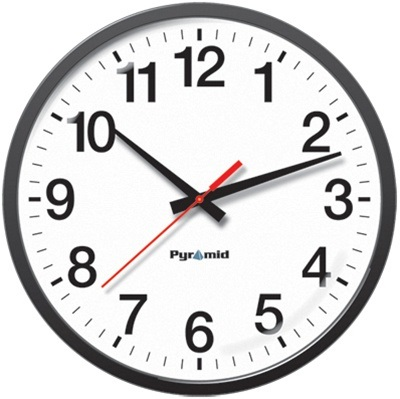In 3rd grade, students are expected to: • Tell and write time to the nearest minute • Use analog and digital clocks to tell time • Measure time intervals in minutes • Solve word problems that involve addition and subtraction…For information on using an area model to represent fractions, visit: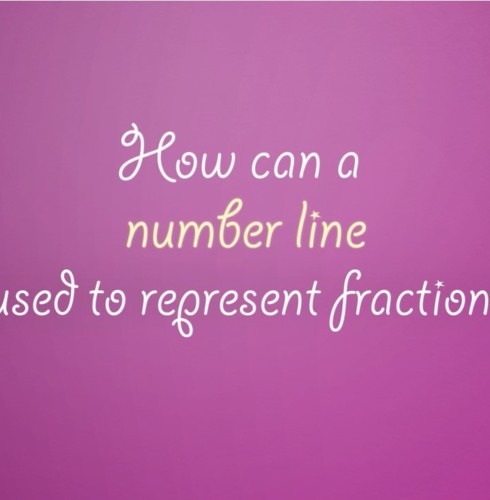For information on representing fractions on a number line visit:In 3rd grade students are expected to, “Understand a fraction as the quantity formed by 1 part when a whole is partitioned into equal parts.” The best way for students to develop this understanding is by putting fractions into real…1. What’s included when teaching area in 3rd grade? • Solving real-world problems involving area of polygons • Solve Real World Problems - Determining when it is necessary to find the area or perimeter from a given story problem. •…Modeling Fractions on a Number Line! In 3rd grade students are expected to, “Understand a fraction as a number on the number line; represent fractions on a number line.” This is a concept that students struggle with if you don’t…In 3rd grade students are developing understanding of fractions. Previously in 1st & 2nd grade they have decomposed shapes into equal parts and described them using fraction language, but in 3rd grade is the first year they represent the fractions…What is the associative property of multiplication? The associative property of multiplication is “an extension of the commutative property; to change the order and group two factors to find convenient products (such as 10) in order to make the multiplication…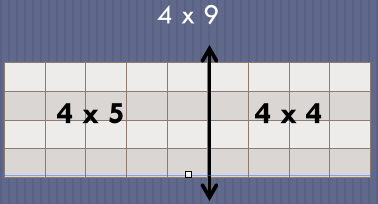Modeling the Distributive Property of Multiplication. In 3rd grade: • students explore, discover and understand the distributive property • are not required to demonstrate understand in the context of a story problem • are limited to factors 0-10 • are…What attributes should we use to describe quadrilaterals? Define shapes using the following attributes: number of sides, number of angles, whether the shape has a right angle, whether the sides are the same length, and whether the sides are straight…Here are the top 5 things you need to consider when teaching or revisiting Geometry concepts in 3rd grade. 1. What is included in the Geometry domain for 3rd grade? • Understand that shapes in different categories may share attributes.…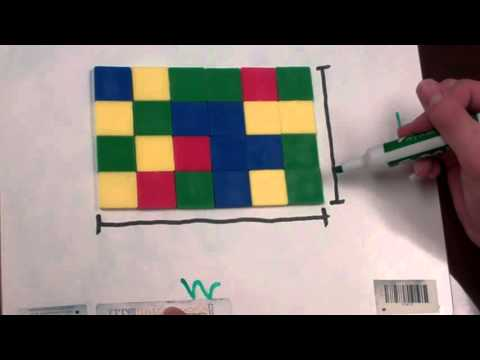1. What’s included when teaching perimeter in 3rd grade? • Solving real-world problems involving perimeter of polygons • Determining when it is necessary to find the perimeter from a given story problem • Finding the perimeter given the side lengths…